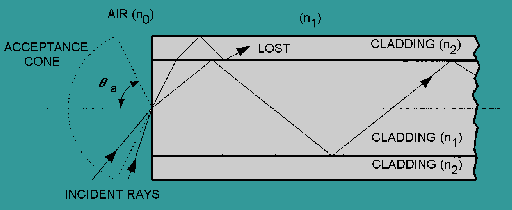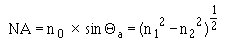propagation of light along a fiber. The ray theory is used to approximate the light acceptance and guiding properties of optical fibers. According to the second theory, light is described as an electromagnetic wave."> Propagation of light along a fiberCustom SearchPROPAGATION OF LIGHT ALONG A FIBER The concept of light propagation, the transmission of light along an optical fiber, can be described by two theories. According to the first theory, light is described as a simple ray. This theory is the ray theory, or geometrical optics, approach. The advantage of the ray approach is that you get a clearer picture of the propagation of light along a fiber. The ray theory is used to approximate the light acceptance and guiding properties of optical fibers. According to the second theory, light is described as an electromagnetic wave. This theory is the mode theory, or wave representation, approach. The mode theory describes the behavior of light within an optical fiber. The mode theory is useful in describing the optical fiber properties of absorption, attenuation, and dispersion. These fiber properties are discussed later in this chapter. Q.21 Light transmission along an optical fiber is described by two theories. Which theory is used to approximate the light acceptance and guiding properties of an optical fiber? Ray Theory Two types of rays can propagate along an optical fiber. The first type is called meridional rays. Meridional rays are rays that pass through the axis of the optical fiber. Meridional rays are used to illustrate the basic transmission properties of optical fibers. The second type is called skew rays. Skew rays are rays that travel through an optical fiber without passing through its axis. MERIDIONAL RAYS. - Meridional rays can be classified as bound or unbound rays. Bound rays remain in the core and propagate along the axis of the fiber. Bound rays propagate through the fiber by total internal reflection. Unbound rays are refracted out of the fiber core. Figure 2-10 shows a possible path taken by bound and unbound rays in a step-index fiber. The core of the step-index fiber has an index of refraction n1. The cladding of a step-index has an index of refraction n2, that is lower than n1. Figure 2-10 assumes the core-cladding interface is perfect. However, imperfections at the core-cladding interface will cause part of the bound rays to be refracted out of the core into the cladding. The light rays refracted into the cladding will eventually escape from the fiber. In general, meridional rays follow the laws of reflection and refraction. Figure 2-10. - Bound and unbound rays in a step-index fiber.It is known that bound rays propagate in fibers due to total internal reflection, but how do these light rays enter the fiber? Rays that enter the fiber must intersect the core-cladding interface at an angle greater than the critical angle (Θc). Only those rays that enter the fiber and strike the interface at these angles will propagate along the fiber. How a light ray is launched into a fiber is shown in figure 2-11. The incident ray I1 enters the fiber at the angle Θa. I1 is refracted upon entering the fiber and is transmitted to the core-cladding interface. The ray then strikes the core-cladding interface at the critical angle (Θ c). I1 is totally reflected back into the core and continues to propagate along the fiber. The incident ray I2 enters the fiber at an angle greater than Θa. Again, I2 is refracted upon entering the fiber and is transmitted to the core-cladding interface. I2 strikes the core-cladding interface at an angle less than the critical angle (Θc). I2 is refracted into the cladding and is eventually lost. The light ray incident on the fiber core must be within the acceptance cone defined by the angle Θa shown in figure 2-12. Angle Θa is defined as the acceptance angle. The acceptance angle (Θa) is the maximum angle to the axis of the fiber that light entering the fiber is propagated. The value of the angle of acceptance (Θa) depends on fiber properties and transmission conditions. Figure 2-11. - How a light ray enters an optical fiber.Figure 2-12. - Fiber acceptance angle.The acceptance angle is related to the refractive indices of the core, cladding, and medium surrounding the fiber. This relationship is called the numerical aperture of the fiber. The numerical aperture (NA) is a measurement of the ability of an optical fiber to capture light. The NA is also used to define the acceptance cone of an optical fiber. Figure 2-12 illustrates the relationship between the acceptance angle and the refractive indices. The index of refraction of the fiber core is n1. The index of refraction of the fiber cladding is n2. The index of refraction of the surrounding medium is n0. By using Snell's law and basic trigonometric relationships, the NA of the fiber is given by:Since the medium next to the fiber at the launching point is normally air, n0 is equal to 1.00. The NA is then simply equal to sin Θa. The NA is a convenient way to measure the light-gathering ability of an optical fiber. It is used to measure source-to-fiber power-coupling efficiencies. A high NA indicates a high source-to-fiber coupling efficiency. Source-to-fiber coupling efficiency is described in chapter 6. Typical values of NA range from 0.20 to 0.29 for glass fibers. Plastic fibers generally have a higher NA. An NA for plastic fibers can be higher than 0.50. In addition, the NA is commonly used to specify multimode fibers. However, for small core diameters, such as in single mode fibers, the ray theory breaks down. Ray theory describes only the direction a plane wave takes in a fiber. Ray theory eliminates any properties of the plane wave that interfere with the transmission of light along a fiber. In reality, plane waves interfere with each other. Therefore, only certain types of rays are able to propagate in an optical fiber. Optical fibers can support only a specific number of guided modes. In small core fibers, the number of modes supported is one or only a few modes. Mode theory is used to describe the types of plane waves able to propagate along an optical fiber. SKEW RAYS. - A possible path of propagation of skew rays is shown in figure 2-13. Figure 2-13, view A, provides an angled view and view B provides a front view. Skew rays propagate without passing through the center axis of the fiber. The acceptance angle for skew rays is larger than the acceptance angle of meridional rays. This condition explains why skew rays outnumber meridional rays. Skew rays are often used in the calculation of light acceptance in an optical fiber. The addition of skew rays increases the amount of light capacity of a fiber. In large NA fibers, the increase may be significant. Figure 2-13. - Skew ray propagation: A. Angled view; B. Front view.The addition of skew rays also increases the amount of loss in a fiber. Skew rays tend to propagate near the edge of the fiber core. A large portion of the number of skew rays that are trapped in the fiber core are considered to be leaky rays. Leaky rays are predicted to be totally reflected at the core-cladding boundary. However, these rays are partially refracted because of the curved nature of the fiber boundary. Mode theory is also used to describe this type of leaky ray loss. Q.22 Meridional rays are classified as either bound or unbound rays. Bound rays propagate through the fiber according to what property? Q.23 A light ray incident on the optical fiber core is propagated along the fiber. Is the angle of incidence of the light ray entering the fiber larger or smaller than the acceptance angle (Θa) Q.24 What fiber property does numerical aperture (NA) measure? Q.25 Skew rays and meridional rays define different acceptance angles. Which acceptance angle is larger, the skew ray angle or the meridional ray angle?Integrated Publishing, Inc. - A (SDVOSB) Service Disabled Veteran Owned Small Business